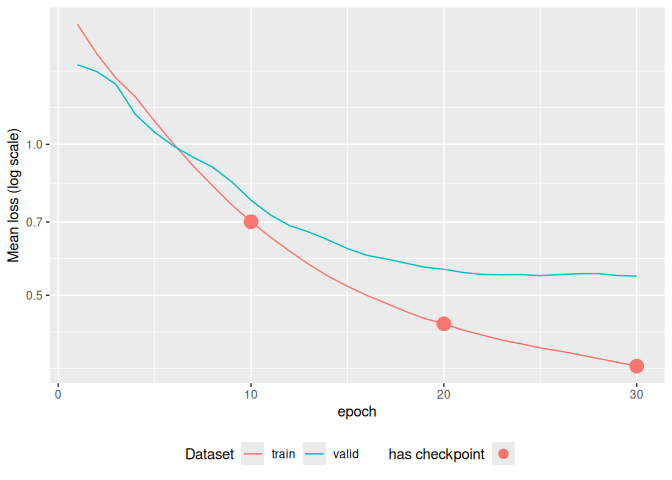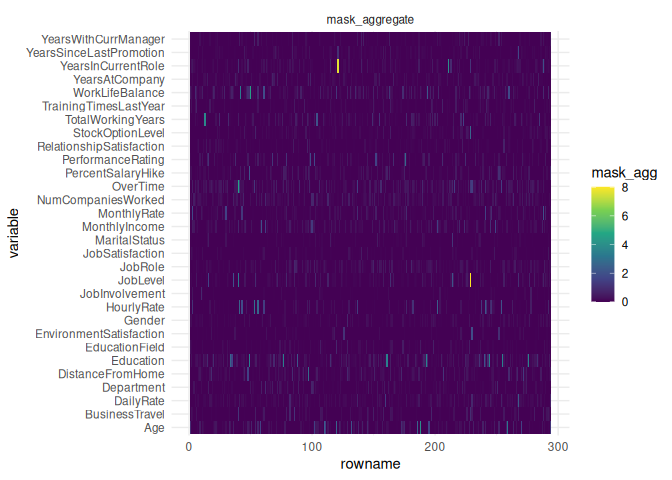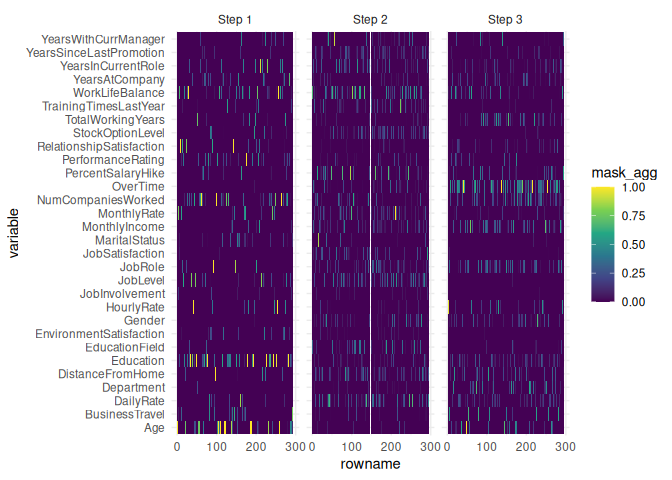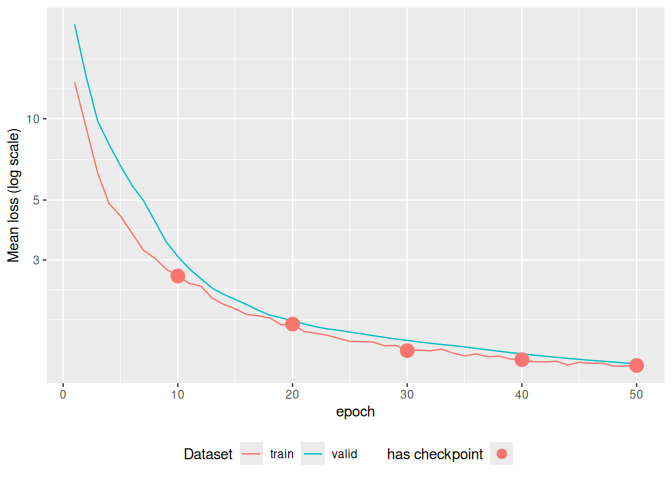An R implementation of: TabNet: Attentive Interpretable Tabular Learning. The code in this repository is an R port of dreamquark-ai/tabnet PyTorch’s implementation using the torch package. The original repository is augmented with Coherent Hierarchical Multi-label Classification Networks

## Installation

You can install the released version from CRAN with:

install.packages("tabnet")

The development version can be installed from GitHub with:

# install.packages("remotes")
remotes::install_github("mlverse/tabnet")

## Basic Binary Classification Example

Here we show a binary classification example of the attrition dataset, using a recipe for dataset input specification.

library(tabnet)
suppressPackageStartupMessages(library(recipes))
library(yardstick)
library(ggplot2)
set.seed(1)

data("attrition", package = "modeldata")
test_idx <- sample.int(nrow(attrition), size = 0.2 * nrow(attrition))

train <- attrition[-test_idx,]
test <- attrition[test_idx,]

rec <- recipe(Attrition ~ ., data = train) %>%
step_normalize(all_numeric(), -all_outcomes())

fit <- tabnet_fit(rec, train, epochs = 30, valid_split=0.1, learn_rate = 5e-3)
autoplot(fit)The plots gives you an immediate insight about model overfitting, and if any, the available model checkpoints available before the overfitting

Keep in mind that regression as well as multi-class classification are also available, and that you can specify dataset through data.frame and formula as well. You will find them in the package vignettes.

## Model performance results

As the standard method predict() is used, you can rely on your usual metric functions for model performance results. Here we use {yardstick} :

metrics <- metric_set(accuracy, precision, recall)
cbind(test, predict(fit, test)) %>%
metrics(Attrition, estimate = .pred_class)
#> # A tibble: 3 × 3
#>   .metric   .estimator .estimate
#>   <chr>     <chr>          <dbl>
#> 1 accuracy  binary         0.837
#> 2 precision binary         0.837
#> 3 recall    binary         1

cbind(test, predict(fit, test, type = "prob")) %>%
roc_auc(Attrition, .pred_No)
#> # A tibble: 1 × 3
#>   .metric .estimator .estimate
#>   <chr>   <chr>          <dbl>
#> 1 roc_auc binary         0.554

## Explain model on test-set with attention map

TabNet has intrinsic explainability feature through the visualization of attention map, either aggregated:

explain <- tabnet_explain(fit, test)
autoplot(explain)or at each layer through the type = "steps" option:

autoplot(explain, type = "steps")## Self-supervised pretraining

For cases when a consistent part of your dataset has no outcome, TabNet offers a self-supervised training step allowing to model to capture predictors intrinsic features and predictors interactions, upfront the supervised task.

pretrain <- tabnet_pretrain(rec, train, epochs = 50, valid_split=0.1, learn_rate = 1e-2)
autoplot(pretrain)The exemple here is a toy example as the train dataset does actually contain outcomes. The vignette on Self-supervised training and fine-tuning will gives you the complete correct workflow step-by-step.

## Missing data in predictors

{tabnet} leverage the masking mechanism to deal with missing data, so you don’t have to remove the entries in your dataset with some missing values in the predictors variables.

# Comparison with other implementations

Group Feature {tabnet} dreamquark-ai fast-tabnet
Input format dataframe
formula
recipe
Node
missings in predictor
Output format dataframe
workflow